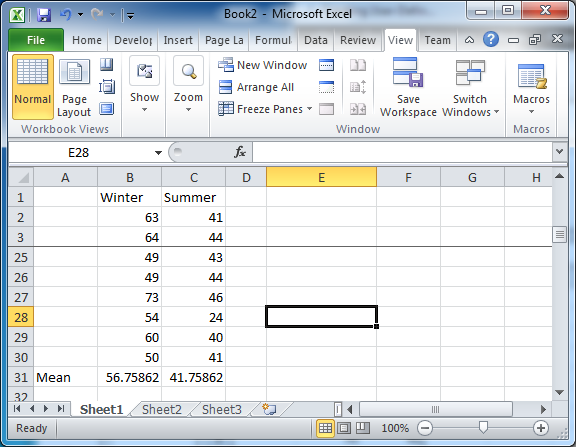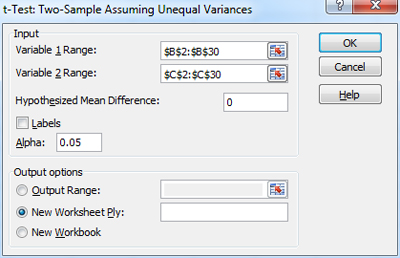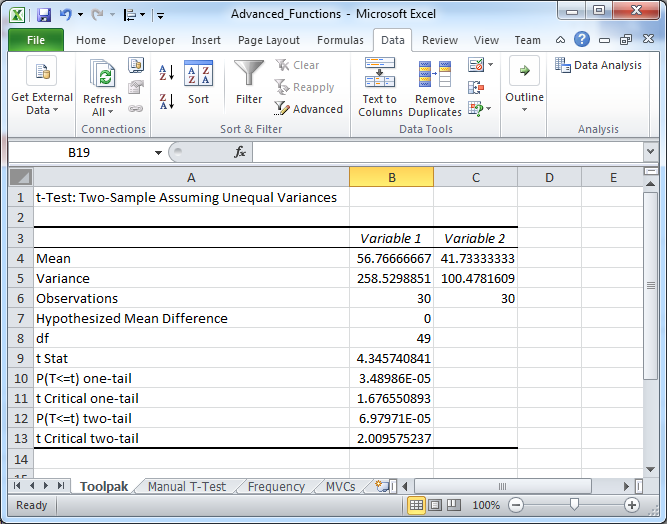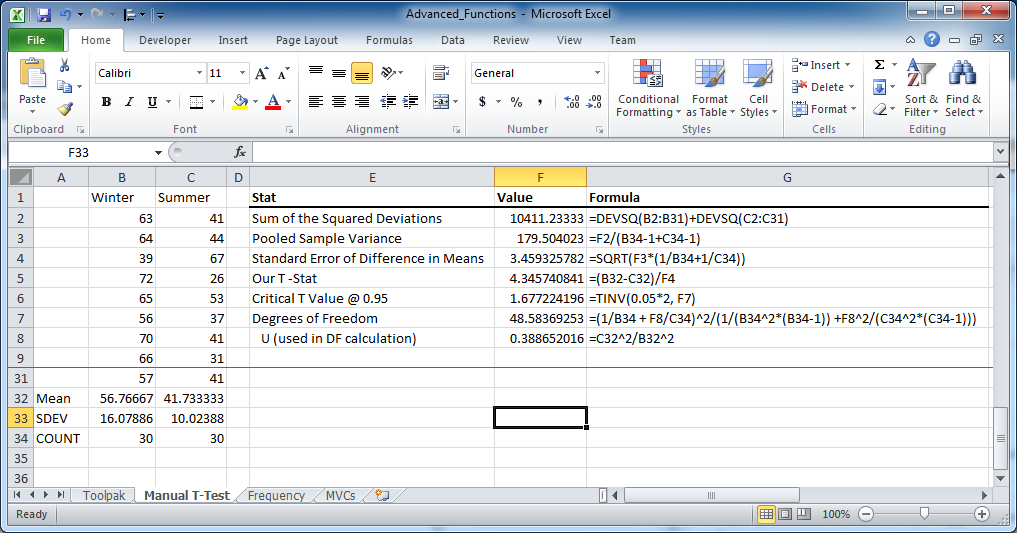### Performing a Statistical T-Test in Excel

The value of the t-Test is that it can tell you if a difference you are seeing in two measurements is legitimate or if the difference is likely to be just from randomness. In this blog post I am going to use the t-Test to determine if the difference in the number of daily motor vehicle collisions during the winter is meaningfully different then the number during the summer. While this is kind of a no-brainer example it will help illustrate the value of the t-Test and give you some ideas on how you might apply it to give some statistical rigour to your analytical findings.

Before we get into the test let me provide some background to help understand what it is we’re trying to do. In statistics we are always comparing two hypotheses. The first is named the null hypothesis and the second is named the alternate hypothesis. We almost always want to reject the null hypothesis and accept the alternate one because in that situation it means something interesting has occurred. For our motor vehicle collision example we want to determine if there is a meaningful difference in the number of daily motor vehicle collisions during the first quarter of the year (i.e. the winter) versus the third quarter of the year (i.e. the summer). Now, you may be thinking “obviously there’s a difference, the weather” but let’s, for the sake of education, assume that we need to demonstrate statistically that there is a difference. In this study the null hypothesis would be that “there is no difference between the quarters, the  number of collisions are the same” while the alternate hypothesis would be “yes, there is a statistically significant difference between the two quarters”.

The term “statistically significant” needs some explanation. When we want something to be statistically significant we want to be able to say, with a particular level of confidence, that the results we are seeing are not just due to chance. First we pick our “confidence level” or how sure we want to be and then, through the magic of statistics, we are provided a number that our results have to beat in that we can be that sure. In this example we’re going with the 95% confidence level which means that if we find a difference between the average number of MVCs during the winter and summer we want to be 95% sure it’s not due to chance. Or, in other words, we are willing to wrongly reject the null hypothesis only 5% of the time. We can up the confidence interval to 99% if we want but, as we’ll see, we’ll need to adhere to even stricter conditions. Whatever we choose just remember that the “significant” part in statistically significant doesn’t mean “important” (as significant typically means in everyday conversation) it just means “not due to randomness”.

How do we go about demonstrating that the two quarters have real, non-random differences in the number of daily motor vehicle collisions? The first thing we do randomly select from the first and third quarters 30 different days worth of MVC counts. The screen grab below shows my randomly selected MVC data.Notice that we there are two columns of data, one marked Winter and one marked Summer and each has 30 entries. Also notice that at the bottom of each column is a number labeled “Mean”. In those cells I have used Excel’s AVERAGE function to find the mean of all the numbers (add them all up, divide by 30). And behold, the means are different. That proves the quarters are different, right? Not quite. We still have to deal with the peskiness that is statistical significance. It’s possible, after all, that it’s just through chance those means are different. As discussed above we want to be 95% sure that they aren’t.

To prolong your agony I’m actually going to show you two ways to perform the t-Test: the (relatively) quick way using Microsoft’s Data Analysis ToolPak Add-in and the longer, manual way that uses built in Excel functions.

The Analysis ToolPak is a free Add-In provided by Microsoft as part of the default installation of Excel. Add-Ins are a kind of Excel file, typically with a .xlam extension, that package together a bunch of related functionality. To get started with the Analysis ToolPak Add-In we need to check if it is installed. You may or may not have it already installed but to check if you do you click the “Data” tab in Excel and look at the far right. If you see “Data Analysis” as an option, it’s already active (see screen shot).If you don’t see it that means we need to turn it on. First, click the green “File’”tab (or the Excel jewel if you’re in 2007) to call up the Save/Open/Close menu. Look around the option named “Options’”and click it. This will open up a window with a menu on the left. Click on the menu option named “Add-Ins” on the left hand side. The screen will change and at the bottom, beside the word “Manage”, will be a select box and a button labeled“Go”. Click the“Go”button to open yet another window and you will see a series of check- boxes. Fingers crossed that one of them says “Analysis ToolPak” and is unchecked. Click the checkbox and click ok. Now go back to Data tab, look at the far right and you’ll see “Data Analysis’”. You’re set.

Now that the ToolPak is installed click the “Data Analysis” button and a modal window will open up. Click “t-Test: Two-Sample Assuming Unequal Variances” in the list and click the “OK” button and this will open up a new window (check the screen shot below) with some options. For the “Variable 1 Range” click the little arrow button and select all of the “Winter” data, which is in B2:31. For “Variable 2 Range” select all the “Summer” data from C2:C31. For the “Hypothesized Mean Difference” enter 0 (since we’re trying to prove they are the same, the difference of the mean should be 0) and everything else can stay the same. However, notice that there is field named “Alpha” that has the value 0.05. It’s not a coincidence that 0.05 = 1 – 0.95. Alpha is another way of asking how sure we want to be.Click the “OK” button and Excel will open up a new worksheet populated with a bunch of labels and values just like the one in the screen shot below.So what are we looking at? Well, there are the means we saw. Next comes the variances, which are measures of how spread out the values are, and they are quite different (which is why we needed to use the test that assumed unequal variances) and a little further down we see our “t Stat” and it has a value of about 4.35. Skip down two lines to the value labeled “t Critical one-tail” and note that it is about 1.68. That’s good news for us because the rule is that if your “t Stat” is larger than your “t Critical” value then the null hypothesis can be rejected and our results are therefore statistically significant. This means that we can now state, with the power of math backing us up, that we are 95% sure that the daily average number of MVCs in the winter are greater than the daily average number of MVCs in the summer.

Second, the manual way.

So we used a built-in tool to do a bunch of heavy lifting for us (as tools should) and we read an answer off a table. It works, but how did it work? How can we calculate these numbers if the ToolPak isn’t around but we still, for some reason, have access to Excel?

First, have a look at this screen shot because it will help to explain each of the steps I used to perform a manual unequal variance t-Test.On the left are the two original columns of data, one for winter and one for summer. Notice that there are 30 entries for each season but that I used “freeze panes” so that I can show the bottom of the data (starting in row 32). For both seasons I calculated three additional variables, the mean (using Excel’s AVERAGE function), the standard deviation (using Excels SDEV function) and the count of the number of data points (using Excel’s COUNT function). These will all be needed in the coming calculations.

On the right hand side of the screen shot I produced my own little table and I’m going to walk through each of these variables and explain the thinking behind them.

The first needed variable is the Sum of the Squared Deviations for which I used Excel’s DEVSQ function as a short cut. Think of the variable like this: you have a mean value for  a season and you have 30 different data points that are either going to be a little bit more or a little bit less than the average (as data points usually are). For each data point, subtract its value from the mean and square it. Now sum those squared differences all up and that’s the sum of the squared deviations (or differences). Notice that I added together the sum of the squared deviations for both the winter and summer seasons together into a single value.

Why did we need the sum of the squared deviations? Because we want to calculate the pooled sample variance. Why pooled? Because we put both the winter and the summer values together. But what’s variance? The average of the squared differences from the mean. This is easy to calculate, just divide the sum of the squared deviations by the number of points we have in our two seasons, minus 2 (1 for each season) to account for Bessel’s correction which is a correction for the bias in the estimation of the population variance (just trust me, subtract 2, Excel does).

The next variable is the Standard Error of Difference in Means and to calculate it we take the square root of the pooled sample variance times the sum of one over the counts for the winter and summer data points (i.e. 1/30 + 1/30). But what is the standard error of difference in means? It’s just the average expected difference between the means for two samples that have this many data points. We’re trying to determine, after all, if two means are significantly different from one another and, given the size of the two sample groups, we should expect that there would be some difference. Now, imagine that we run this little study over and over again with other, randomly selected groups of MVCs, each pair would have their own difference between the means. That sounds like a lot of work, so instead of running this study over and over again we can instead use the formula for the standard error of difference to answer the question: “what’s the expected average of that difference?”.

But what do we need that for? To calculate our t-statistic. And to perform that calculation we just divide the actual difference in the means (winter mean minus summer mean) by the standard error of difference in means to end up with approximately 4.35, just like the ToolPak did.

But we’re not done, we still need to determine our critical t-score at the 95% confidence interval. To do that we cheat a bit and use Excel’s built in TINV function. The first input is the probability that we want to test, in this case it’s 0.05 (i.e. 1-0.95) or the alpha from the ToolPak. The eagle-eyed will note that in the calculation in the screen shot 0.05 is actually multiplied by 2 and this is because we’re interested in replicating the “one-tailed” result from the ToolPak and since TINV is “two-tailed” we need to double up the probability to account for that.

TINV also takes a second parameter, degrees of freedom, and usually degrees of freedom is pretty straightforward (it’s just the sum of the two counts, minus 2, like for the pooled variance) except when we’re dealing with an unequal variance scenario. In order to handle this scenario we need to break out the more elaborate calculation defined by Moser and Stevens 1992 and spelled out in the ugly Excel formula:

=(1/B34 + F8/C34)^2/(1/(B34^2*(B34-1)) + F8^2/(C34^2*(C34-1)))

Where the value in cell F8 is calculated as =C32^2/B32^2 or the ratio of the squared standard deviations for each season. God, it’s a mess, I know. But just dissect the formula and you’ll see that it’s just a complicated formula using the counts for the two seasons in multiple places. One thing you may notice is that the resulting degrees of freedom is not an integer and that we have the approximate value 48.58. The ToolPak results above have a degrees of freedom of 49. This is because Microsoft rounds. You can choose to round or not, the results are pretty much the same.

With degrees of freedom we can finally run the TINV function and when we do we get a critical t-stat of 1.68, just like the ToolPak (although the ToolPak number is slightly different further into the decimals because I didn’t round my degrees of freedom). And just like with the ToolPak, our t-stat is way bigger than that critical t-value so we can reject the null hypothesis and assume that we have statistically significant results at the 95% confidence level. Yes!

And with that we are finally done our odyssey of manually calculating the unequal variance t-test. We’ve calculated the same results as the ToolPak and we got right into the nitty-gritty of it. Well done.

Conclusion

If you made it this far (and didn’t just skip the manual section) then bravo, that was a real grind. Either way, I hope you learned something about the t-statistic and how it can be used to add some rigour to pronouncements about means. I do, somewhat unfortunately, find that a lot of the time the t-stat plays spoiler in that decision makers see a difference in averages and conclude that something is working when the reality is that, statistically speaking, there is no significant difference. Nevertheless, it’s important to be able to back up your analysis with some math and t-stats are a great way to provide that oomph. Good luck.